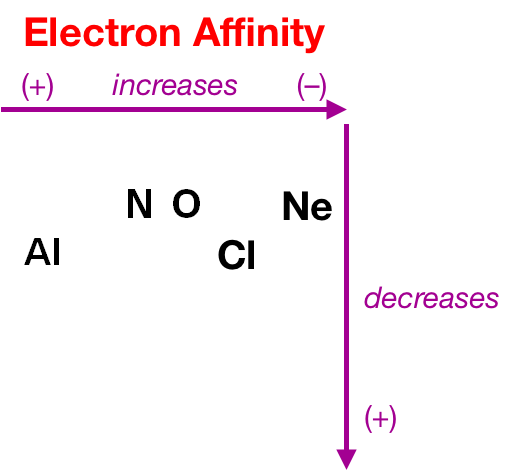# Problem: Which electron affinity below would you predict to be the most exothermic?(1) Al(g) + e− → Al–(g)(2) N(g) + e− → N−(g)(3) Ne(g) + e− → Ne−(g)(4) Cl(g) + e− → Cl−(g)(5) O−(g) + e− → O2−(g)

###### FREE Expert Solution

Recall that electron affinity is the energy change from the addition of 1 e to a gaseous element/ion

Atom(g) + e  Ion(g), ΔE = –E.A. → more negative → most exothermic

It increases from left to right and decreases down a period in the periodic table.###### Problem Details

Which electron affinity below would you predict to be the most exothermic?

(1) Al(g) + e → Al(g)

(2) N(g) + e → N(g)

(3) Ne(g) + e− → Ne(g)

(4) Cl(g) + e → Cl(g)

(5) O(g) + e → O2(g)